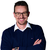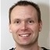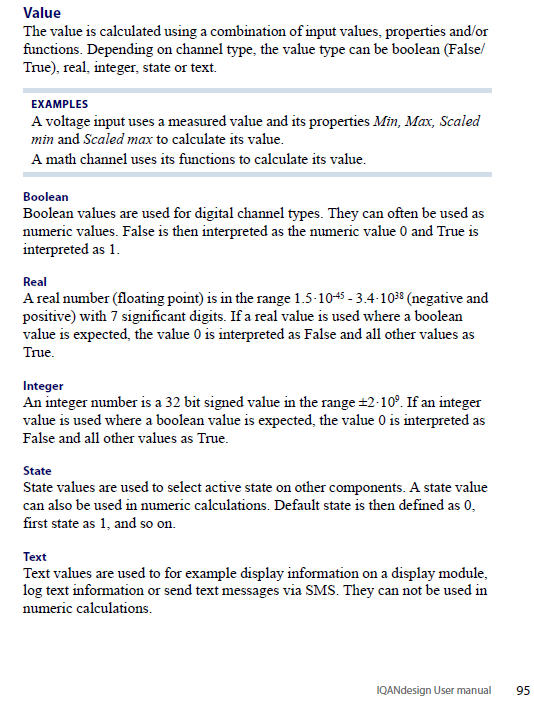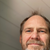+1

# Integer vs. real values

Arno Heeren 7 years ago in IQANdesign updated by Ulrik Zakariasson (Software development) 6 years ago
Hi,
the answer might be interesting also for other users:
When should I use integer and when real values?
CAN-communication is one example where I see benefits using integer values.
Thanks.GOOD, I'M SATISFIED
Satisfaction mark by Arno Heeren 6 years ago+2
Hi Arno, thanks for an interesting question.

For most of your channels, e.g. when calculating the command to a proportional output, you should use Real values, as this gives you more precision, without adding any significant cost in calculation performance.
All modern IQAN master modules (those on the IQANdesign platform) have processors that handles 32-bit floating point numbers.

There are only a few cases I can think of when you actually have to use integers.
One is in the unusual case that you need to do some bitwise operations. This could become necessary for some CAN messages, but the GPIN/GPOUT introduced in 3.00 has built in functions for handling the more common cases, such as reversing byte order.
Note that if you are performing bitwise operations, that the Integer type we use in IQAN is a 32-bit signed. In cases where you have a high-precision signal sent on CAN, it is often represented as a 32-bit unsigned, with some scaling. Then it is best to use the JPIN/JPOUT.

For the J1939 CAN channels JPIN/JPOUT, you will automatically work with real values if that is needed, as the J1939 scaling and offset is built in as properties, and change value type depending on these.

Another case where you have to select an channel of integer type is if you use this channel to determine a state, e.g. using the IMAC as input channle to the SP channel.

A third case that I also think is extremely unusual for machine conrol is if you calculate a very large value where you need accuracy with >7 signifcant digits.Then there are cases where using an integer gives a slightly nicer design. For example if you have a paramter that is adjusted in steps of one, you could use the IP channel instead of the FP channel for this. The visual difference is that when adjusting in the menu systemyou will see the value change from 1, 2, 3 and so on instead of 1.00, 2.00, 3.00.